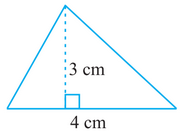# Q. 2.     Find the area of each of the following triangles:R Riya

We know that

Area of triangle

Here,

Base of triangle = 4 cm

and

Height of triangle =3 cm

Therefore, area of triangle is

Exams
Articles
Questions